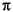MATLAB Function Referencelogm

Matrix logarithm

Syntax

• ```L =` `logm(A)
```[L, exitflag] = logm(A)
``````

Description

```L = logm(A) ``` is the principal matrix logarithm of A, the inverse of `expm(A)`. `L` is the unique logarithm for which every eigenvalue has imaginary part lying strictly between -and. If `A` is singular or has any eigenvalues on the negative real axis, the principal logarithm is undefined. In this case, `logm` computes a non-principal logarithm and returns a warning message.

`[L, exitflag] = logm(A)` returns a scalar `exitflag` that describes the exit condition of `logm`:

• If `exitflag = 0`, the algorithm was successfully completed.
• If `exitflag = 1`, one or more Taylor series evaluations did not converge. However, the computed value of `L` might still be accurate.

The input `A` can have class `double` or `single`.

Remarks

If `A` is real symmetric or complex Hermitian, then so is `logm(A)`.

Some matrices, like `A = [0 1; 0 0]`, do not have any logarithms, real or complex, so `logm` cannot be expected to produce one.

Limitations

For most matrices:

• ```logm(expm(A)) = A = expm(logm(A))
```

These identities may fail for some `A`. For example, if the computed eigenvalues of `A` include an exact zero, then `logm(A)` generates infinity. Or, if the elements of `A` are too large, `expm(A)` may overflow.

Examples

Suppose `A` is the 3-by-3 matrix

• ```        1        1        0
0        0        2
0        0       -1
```

and `Y =` `expm(A)` is

• ```Y =
`    `2.7183`    `1.7183`    `1.0862
`         `0`    `1.0000`    `1.2642
`         `0`         `0`    `0.3679
```

Then `A = logm(Y)` produces the original matrix `A`.

• ```Y` `=
`    `1.0000`    `1.0000`    `0.0000
`         `0`         `0`    `2.0000
`         `0`         `0`   `-1.0000
```

But `log(A)` involves taking the logarithm of zero, and so produces

• ```ans =
`    `1.0000`    `0.5413`    `0.0826
`      `-Inf`         `0`    `0.2345
`      `-Inf`      `-Inf`   `-1.0000
```

Algorithm

The algorithm `logm` uses is described in .

See Also

`expm`, `funm`, `sqrtm`

References

  Davies, P. I. and N. J. Higham, "A Schur-Parlett algorithm for computing matrix functions," SIAM J. Matrix Anal. Appl., Vol. 25, Number 2, pp. 464-485, 2003.

  Cheng, S. H., N. J. Higham, C. S. Kenney, and A. J. Laub, "Approximating the logarithm of a matrix to specified accuracy," SIAM J. Matrix Anal. Appl., Vol. 22, Number 4, pp. 1112-1125, 2001.

  Higham, N. J., "Evaluating Pade approximants of the matrix logarithm," SIAM J. Matrix Anal. Appl., Vol. 22, Number 4, pp. 1126-1135, 2001.

  Golub, G. H. and C. F. Van Loan, Matrix Computation, Johns Hopkins University Press, 1983, p. 384.

  Moler, C. B. and C. F. Van Loan, "Nineteen Dubious Ways to Compute the Exponential of a Matrix," SIAM Review 20, 1979,pp. 801-836.

© 1994-2005 The MathWorks, Inc.